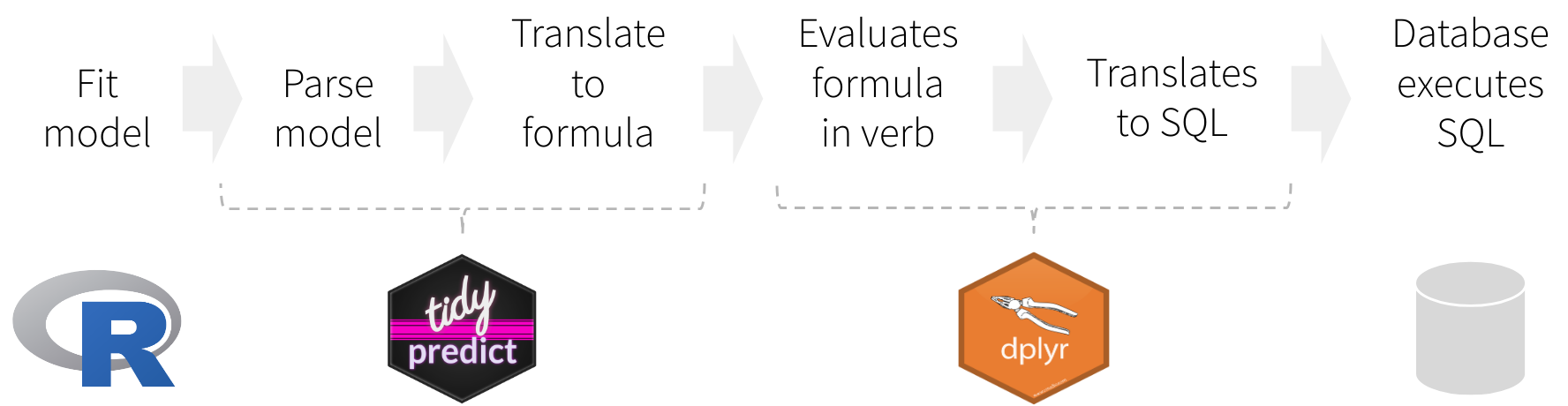The main goal of tidypredict is to enable running predictions inside databases. It reads the model, extracts the components needed to calculate the prediction, and then creates an R formula that can be translated into SQL. In other words, it is able to parse a model such as this one:

model <- lm(mpg ~ wt + cyl, data = mtcars)

tidypredict can return a SQL statement that is ready to run inside the database. Because it uses dplyr’s database interface, it works with several databases back-ends, such as MS SQL:

tidypredict_sql(model, dbplyr::simulate_mssql())
## <SQL> 39.6862614802529 + (wt * -3.19097213898374) + (cyl * -1.5077949682598)

## Installation

Install tidypredict from CRAN using:

# install.packages("tidypredict")

Or install the development version using devtools as follows:

# install.packages("remotes")
# remotes::install_github("tidymodels/tidypredict")

## Functions

tidypredict has only a few functions, and it is not expected that number to grow much. The main focus at this time is to add more models to support.

Function Description
tidypredict_fit() Returns an R formula that calculates the prediction
tidypredict_sql() Returns a SQL query based on the formula from tidypredict_fit()
tidypredict_to_column() Adds a new column using the formula from tidypredict_fit()
tidypredict_test() Tests tidyverse predictions against the model’s native predict() function
tidypredict_interval() Same as tidypredict_fit() but for intervals (only works with lm and glm)
tidypredict_sql_interval() Same as tidypredict_sql() but for intervals (only works with lm and glm)
parse_model() Creates a list spec based on the R model
as_parsed_model() Prepares an object to be recognized as a parsed model

## How it worksInstead of translating directly to a SQL statement, tidypredict creates an R formula. That formula can then be used inside dplyr. The overall workflow would be as illustrated in the image above, and described here:

1. Fit the model using a base R model, or one from the packages listed in Supported Models
2. tidypredict reads model, and creates a list object with the necessary components to run predictions
3. tidypredict builds an R formula based on the list object
4. dplyr evaluates the formula created by tidypredict
5. dplyr translates the formula into a SQL statement, or any other interfaces.
6. The database executes the SQL statement(s) created by dplyr

### Parsed model spec

tidypredict writes and reads a spec based on a model. Instead of simply writing the R formula directly, splitting the spec from the formula adds the following capabilities:

1. No more saving models as .rds - Specifically for cases when the model needs to be used for predictions in a Shiny app.
2. Beyond R models - Technically, anything that can write a proper spec, can be read into tidypredict. It also means, that the parsed model spec can become a good alternative to using PMML.

## Supported models

The following models are supported by tidypredict:

• Linear Regression - lm()
• Generalized Linear model - glm()
• Random Forest models - randomForest::randomForest()
• Random Forest models, via ranger - ranger::ranger()
• MARS models - earth::earth()
• XGBoost models - xgboost::xgb.Booster.complete()
• Cubist models - Cubist::cubist()
• Tree models, via partykit - partykit::ctree()

### parsnip

tidypredict supports models fitted via the parsnip interface. The ones confirmed currently work in tidypredict are:

• lm() - parsnip: linear_reg() with “lm” as the engine.
• randomForest::randomForest() - parsnip: rand_forest() with “randomForest” as the engine.
• ranger::ranger() - parsnip: rand_forest() with “ranger” as the engine.
• earth::earth() - parsnip: mars() with “earth” as the engine.

### broom

The tidy() function from broom works with linear models parsed via tidypredict

pm <- parse_model(lm(wt ~ ., mtcars))
tidy(pm)
## # A tibble: 11 x 2
##    term        estimate
##    <chr>          <dbl>
##  1 (Intercept) -0.231
##  2 mpg         -0.0417
##  3 cyl         -0.0573
##  4 disp         0.00669
##  5 hp          -0.00323
##  6 drat        -0.0901
##  7 qsec         0.200
##  8 vs          -0.0664
##  9 am           0.0184
## 10 gear        -0.0935
## 11 carb         0.249